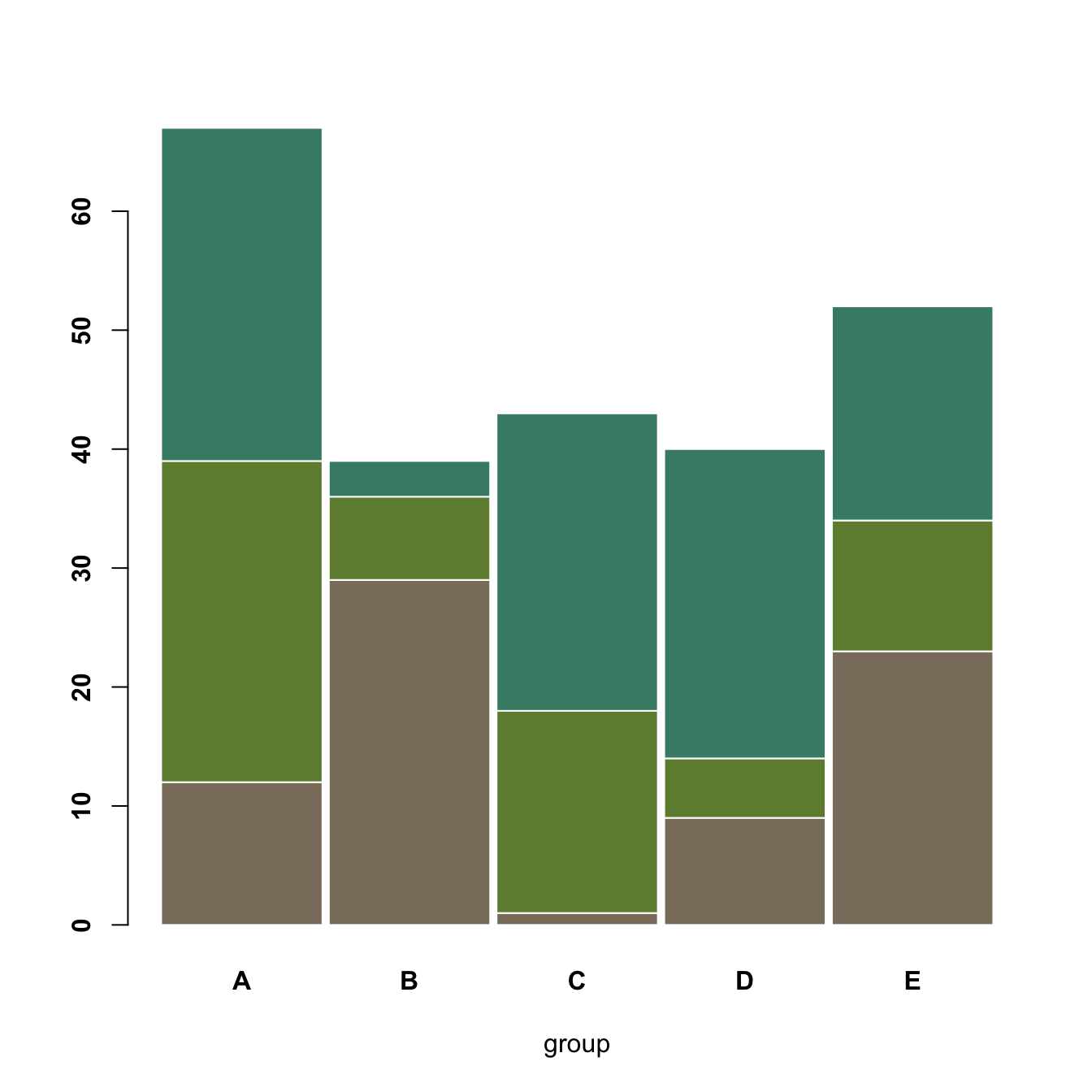# Grouped, stacked and percent stacked barplot in base R

This post explains how to build grouped, stacked and percent stacked barplot with base R. It provides a reproducible example with code for each type.

# Grouped barchart

A grouped barplot display a numeric value for a set of entities split in groups and subgroups. Before trying to build one, check how to make a basic barplot with `R` and `ggplot2`.

A few explanation about the code below:

• input dataset must be a numeric matrix. Each group is a column. Each subgroup is a row.
• the `barplot()` function will recognize this format, and automatically perform the grouping for you.
• the `beside` allows to toggle between the grouped and the stacked barchart``````# Create data
set.seed(112)
data <- matrix(sample(1:30,15) , nrow=3)
colnames(data) <- c("A","B","C","D","E")
rownames(data) <- c("var1","var2","var3")

# Grouped barplot
barplot(data,
col=colors()[c(23,89,12)] ,
border="white",
font.axis=2,
beside=T,
legend=rownames(data),
xlab="group",
font.lab=2)``````

# Grouped barchart

A stacked barplot is very similar to the grouped barplot above. The subgroups are just displayed on top of each other, not beside. The stacked barchart is the default option of the `barplot()` function in base R, so you don’t need to use the `beside` argument.``````# Create data
set.seed(112)
data <- matrix(sample(1:30,15) , nrow=3)
colnames(data) <- c("A","B","C","D","E")
rownames(data) <- c("var1","var2","var3")

# Get the stacked barplot
barplot(data,
col=colors()[c(23,89,12)] ,
border="white",
space=0.04,
font.axis=2,
xlab="group")``````

# Percent stacked barplot

A percent stacked barchart displays the evolution of the proportion of each subgroup. The sum is always equal to 100%.

In base R, you have to manually compute the percentages, using the `apply()` function. This is more straightforward using ggplot2.

Note that here, a custom color palette is used, thanks to the `RColorBrewer` package.``````# Create data
set.seed(1124)
data <- matrix(sample(1:30,15) , nrow=3)
colnames(data) <- c("A","B","C","D","E")
rownames(data) <- c("var1","var2","var3")

# create color palette:
library(RColorBrewer)
coul <- brewer.pal(3, "Pastel2")

# Transform this data in %
data_percentage <- apply(data, 2, function(x){x*100/sum(x,na.rm=T)})

# Make a stacked barplot--> it will be in %!
barplot(data_percentage, col=coul , border="white", xlab="group")``````

Related chart types

## Contact

This document is a work by Yan Holtz. Any feedback is highly encouraged. You can fill an issue on Github, drop me a message on Twitter, or send an email pasting yan.holtz.data with gmail.com.